•#### Learning Bayesian Models with R#### Overview of this book

Learning Bayesian Models with RCreditswww.PacktPub.comPrefaceFree Chapter
Introducing the Probability TheoryThe R EnvironmentIntroducing Bayesian InferenceMachine Learning Using Bayesian InferenceBayesian Regression ModelsBayesian Classification ModelsBayesian Models for Unsupervised LearningBayesian Neural NetworksBayesian Modeling at Big Data ScaleIndex## Model overfitting and bias-variance tradeoff

The expected loss mentioned in the previous section can be written as a sum of three terms in the case of linear regression using squared loss function, as follows: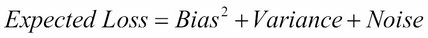Here, Bias is the difference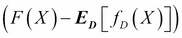between the true model F(X) and average value of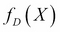taken over an ensemble of datasets. Bias is a measure of how much the average prediction over all datasets in the ensemble differs from the true regression function F(X). Variance is given by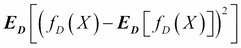. It is a measure of extent to which the solution for a given dataset varies around the mean over all datasets. Hence, Variance is a measure of how much the functionis sensitive to the particular choice of dataset D. The third term Noise, as mentioned earlier, is the expectation of difference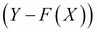between observation and the true regression function, over all the values of X and Y. Putting all these together, we can write the following: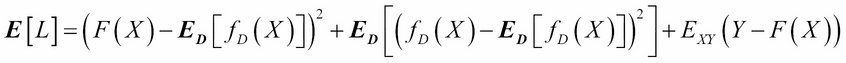The objective of machine learning is to learn the functionfrom data that minimizes...